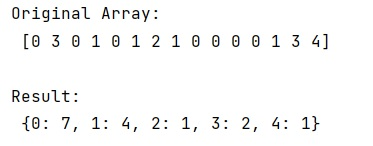# Count the occurrence of all elements in a NumPy ndarray

In this tutorial, we will learn how to count the occurrence of all elements in a NumPy ndarray? By Pranit Sharma Last updated : May 23, 2023

Given a NumPy ndarray, we have to count the occurrence of all elements in it.

## Counting the occurrence of all elements in an ndarray

To count the occurrence of all elements in a NumPy ndarray, we will use numpy.unique() method by passing an argument called return_counts=True and then create a dictionary of unique values and their count so that we can get the count of each element in a ndarray.

Let us understand with the help of an example,

## Python program to count the occurrence of all elements in an ndarray

```# Import numpy
import numpy as np

# Creating a numpy array
arr = np.array([0, 3, 0, 1, 0, 1, 2, 1, 0, 0, 0, 0, 1, 3, 4])

# Getting unique values and their count
un, co = np.unique(arr, return_counts=True)

# Display Original Array
print("Original Array:\n",arr,"\n")

# Combining unique and counts
res = dict(zip(un, co))

# Display Result
print('Result:\n',res)
```

### Output

```Original Array:
[0 3 0 1 0 1 2 1 0 0 0 0 1 3 4]

Result:
{0: 7, 1: 4, 2: 1, 3: 2, 4: 1}
```

### Output (Screenshot)